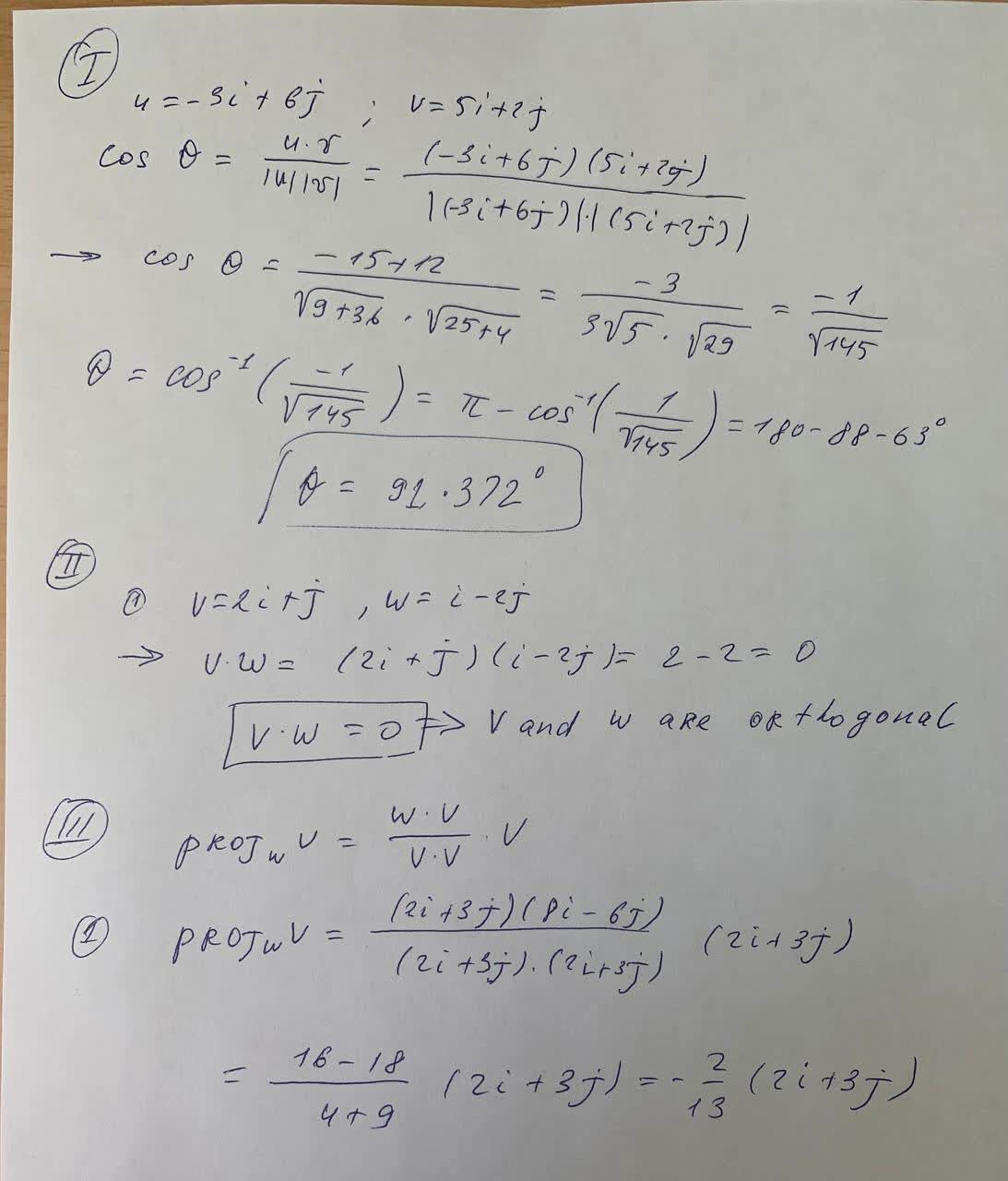#### Didn’t find what you are looking for?

Question# Find the angle between the given vectors . Round to the nearest tenth of a degree. 1) u= -3i +6j ,v=5i + 2j 2) u= i -j , v=2i +3j Use the dot product

Vectors
ANSWEREDFind the angle between the given vectors . Round to the nearest tenth of a degree.
1) u= -3i +6j ,v=5i + 2j
2) u= i -j , v=2i +3j
Use the dot product to determiine wheter the vectors are parallel, orthogonal , or neither .
1) v = 2i +j, w = i-2j
2) v = 4i-j , w=8i-2j
3) v= 3i +3j , w=3i -2j
Find proj w v
1) v = 2i +3j . w = 8i- 6j
2) v= 2i -3j . w =-3i +j